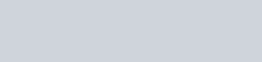A dealer sells an article for Rs. 24 and gains as much
Question:

A dealer sells an article for Rs. 24 and gains as much percent as the cost price of the article. Find the cost price of the article.

Solution:

Let the cost price of article be Rs. x.

Then, gain percent = x

Therefore, the selling price of article

$=\left(x+\frac{x}{100} \times x\right)$

$=\frac{x^{2}+100 x}{100}$

It is given that

$\frac{x^{2}+100 x}{100}=24$

$x^{2}+100 x=2400$

$x^{2}+100 x-2400=0$

$x^{2}+120 x-20 x-2400=0$

$x(x+120)-20(x+120)=0$

$(x+120)(x-20)=0$Because cannot be negative.

Thus, $x=20$ is the require solution.

Therefore, the cost price of article be $x=$ Rs. 20